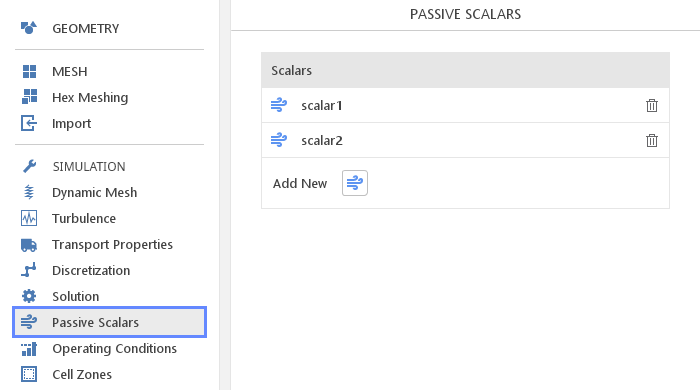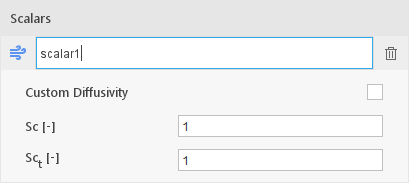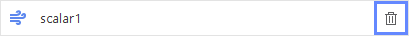# Passive Scalars

## IntroductionThere are situations when one want to simulate transport of some specie in the flow. In general, you would have to employ species transport model. This model is rather complex and only applies to compressible flows, which means you would not be able to use it to for example simulate contamination in the water. In such cases, it might be appropriate to use the Passive Scalars model.

The passive scalar model can be used when changes in scalar concentrations do not influence (hence "passive") the physical properties of the flow like density or viscosity. This condition is usually met when the scalar is used to model relatively small concentrations of some specie (e.g. $$CO_2$$ in air). The equation solved for each scalar $$\Psi$$ is a genera convection-diffusion equation of the form:

$$\frac{\partial \Psi}{ \partial t} + \nabla \cdot (\vec U \Psi) = \nabla \cdot (D \nabla \Psi) + S \Psi$$

where:

• $$\vec U$$ is velocity obtained from momentum equation,

• $$D$$ is diffusivity coefficient and

• $$S \Psi$$ is optional source term.

## Adding New ScalarsYou can add new passive scalars by simply clicking the Add New button below the Scalars list. Newly created scalar will be displayed in the list and will be available in all contexts other equations and fields are available (e.g. boundary and initial conditions).

## Editing ScalarsScalars are created with default name which can be, as usual, changed by double-clicking on the scalar name. Single click on the name will expand options available for a given scalar.

Options:

• Custom Diffusivity – by default the diffussivity coefficient $$D$$ is retrieved from turbulence model but you can override it and manually specify constant Diffusivity

• $$Sc[-]$$ -

• $$Sc_t[-]$$ -

## Removing ScalarsScalars, like other entities, can be removed by simply clicking on the Delete button on the right-hand side of the list.